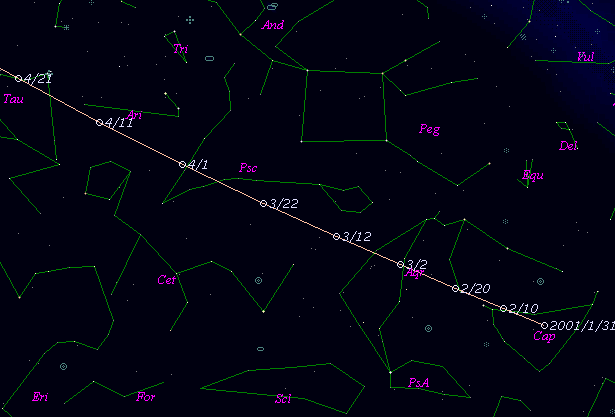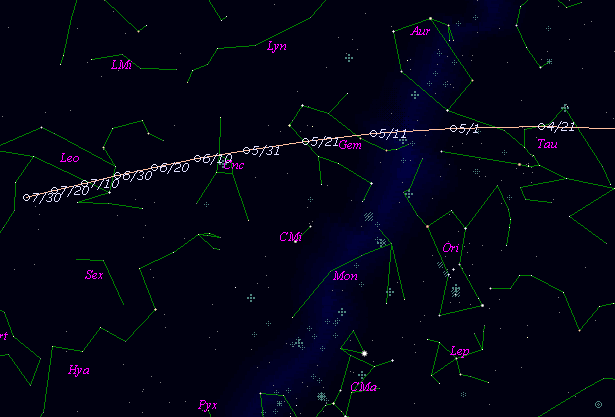# 45P/Honda-Mrkos-Pajdusakova (2001)###Orbital Elements

```   The following improved orbital elements by Kenji Muraoka,
are from 179 observations 1990 to 2001, including 9 Planets,
Moon and 5 minor planets perturbations and non-gravitational
effect of style II. The mean residual is +/- 0.85 arc seconds.

Epoch  =  2001 Apr.  1.0  TT       JDT = 2452000.5
T  =  2001 Mar. 29.92677       +/- 0.00022 (m.e.) TT
Peri. =  326.13195                +/- 0.00039
Node  =   89.08169                +/- 0.00033   (2000.0)
Incl. =    4.25559                +/- 0.00001
q  =    0.5284108              +/- 0.0000005 AU
e  =    0.8250781              +/- 0.0000001
a  =    3.0208383              +/- 0.0000013 AU
n  =    0.18772100             +/- 0.00000012
P  =    5.250                  +/- 0.0000034  years
A1  =   +0.299                  +/- 0.025
A2  =   -0.05531                +/- 0.00005
```

###Finding Charts###Magnitudes Graph

```        m1 = 16.0 + 5 log d + 30.0 log r  [ ,0]  (             - 2001 Mar. 29)
m1 = 12.0 + 5 log d + 15.0 log r  [0, ]  (2001 Mar. 29 -             )
```##### The orbital elements are calculated by Kenji Muraoka. The charts are made with StellaNavigator Ver.2.0 for Windows (AstroArts / ASCII). The magnitudes graphs are made with Comet for Windows.